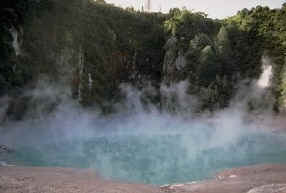# Math Research Projects on Water

I'm going to propose some research projects to my students, addressing issues about water from a mathematical point of view .

The students have just finished 7th grade and are familiar with elementary algebra, geometry and probability. They also have learned basic Pascal programming during last year, so these projects can benefit from computer programming or simulation.

1. What topics do you suggest?
2. What are good references (books, articles, websites) which can be used to define the titles?

• Does issues about water mean water scarcity, pollution, fluid dynamics, behaviour of water molecules, or something else? – Tommi Jun 14 '15 at 7:59
• As @TommiBrander comments, your question is way too broad. Do you mean the supply of water by utilities, the distribution of water in nature, industrial applications of water, etc.? Please be more specific. – Joel Reyes Noche Jun 14 '15 at 10:59
• Maybe this should be obvious, but I am unsure: Does titles refer to topics (i.e., the titles of prospective research projects)? – Benjamin Dickman Jun 15 '15 at 14:11
• @TommiBrander Well Anything related to water which can be though of from a mathematical point of view, is appropriate! – Behzad Jun 16 '15 at 4:50
• @Behzad "Anything related to water" is extraordinarily broad... – Benjamin Dickman Jun 17 '15 at 2:05

Three possible topics:

(1) Tides.

Not easy to understand (it awaited Newton), and somewhat intricate. But you could present some mathematical justification, using, e.g., the discussion here. Once you understand tides, you should be able to convince them that their own weight varies in concert with the moon's tidal pull, and maybe calculate that variation (~$$10$$ mg), from $$F = G \, \frac{m_1 m_2}{r^2}$$ as a project. Make that a goal: Understand tides well enough to realize our weight changes in concert with the tides; and compute that (miniscule) change.(Image from a Jim Brau page.)

(2) Evaporation.

The evaporation rate (at fixed temperature, fixed pressure) is proportional to surface area. At room temperature, with no wind, normal humidity, water evaporates at (very!) roughly $$4 \times 10^{-5} \, \mathrm{kg/s/m}^2$$ (kilograms of water per second per meter squared of surface area). From this one can calculate how long it would take a glass of water to evaporate, or a swimming pool, or a lake:(Image from KidZone.)
Those calculations could form a project: Understand the consequences of evaporation time-linear w.r.t. surface-area, and compute several common instances. (Spherical raindrop evaporation would be quite challenging for 7th-graders.)

(3) Waves.

Generally 7th-graders (in the U.S.) have no exposure to trigonometry, but fortunately ocean waves are not sine waves, but instead better approximated by trochoids, for physical reasons (which one can sense while ocean-bathing):(Image from HyperPhysics.)
I can imagine a project that explores trochoids both geometrically and through computational simulations. There could also be a nice experimental component: measure the speed of water waves (celerity), and predict how long it would take an ocean wave to travel from coast $$A$$ to coast $$B$$—from Cairo to Cyprus, or whichever geographical locations are most salient to the students.

• One could also look at the economics of water, in particular how expensive is it to transport. One can consider water conservation in an environment like the International Space Station, or the expense in supporting a desert outpost with sufficient water needs. Gerhard "California Needs Some More Ideas" Paseman, 2015.06.16 – Gerhard Paseman Jun 17 '15 at 1:55

Students could investigate some simple hydraulics such as the pressure per square inch relationships used to power hydraulic jacks. The calculation of these is simple, staying within the use of proportions and that should be within the abilities of seventh graders. Just learning the calculation of lifting power could be very dry, (pun intended), but the ability to manipulate a very heavy object would get kids' attention. Many theme parks, (notably Disney World) have a sculpture of a large ball, usually made of granite or other HEAVY material. This ball is located in a socket and water is pumped in to the reservoir under the ball effectively lifting the ball on a thin layer of water. Since the friction between the ball and the water has a very small value, it is easy to spin the heavy ball. There could be investigation into the friction coefficients of wet and dry surfaces leading into car safety discussions. Students could also construct a working physical version of this.

Few extra ideas:

1. Operations research wrt sub hunting. Classic essay in World of Mathematics. Very readable. And topic will interest some students.

2. Some simple naval architecture. There are a few simple equations that your students would have the math chops to understand fine (e.g. how max speed is connected to length of ships for non hydroplaning hulls).

3. Economics of fracking (hydraulic fracturing) or water disposal. For each just give them the formulas and let them play with scale. /For the latter there are some simple things to do with percentages or ratios (oil/water/gas).

4. Fluid flow in pipes. Often this is shown with lots of calculus, but the essential relations are algebraic. This is how most of it is taught in the Navy. for instance the power laws of velocity, head loss, and pump power (linear, quadratic, cubic): doubling flow requires 8 times the power. Working engineers often don't even use any algebra but add up head loss by arithmetic and size pumps from the Ingersol Rand catalog. But learning to use a nomograph is a skill itself.

5. Open channel flow. Provide the equation and have students work a few problems doing inputs. (Make realistic if possible, using numbers from codes, real sewer projects or even rivers.) See Michael Lindberg texts for EIT or mechanical PE. (simple formulas, just don't have to hand.)